# A hollow, conducting sphere with an outer radius of 0.255 m and an inner radius of 0.200 m

A hollow, conducting sphere with an outer radius of 0.255 m and an inner radius of 0.200 m has a uniform surface charge density of +6.37 10-6 C/m2. A charge of -0.340 �C is now introduced into the cavity inside the sphere.
(a) What is the new charge density on the outside of the sphere?
(b) Calculate the strength of the electric field just outside the sphere.
( c) What is the electric flux through a spherical surface just inside the inner surface of the sphere?

Concepts and reason
The required concepts to solve this question are surface charge density, electric field, and Gauss law.
Use the relation between charge density and charge to find the new charge density on the outside of the sphere.
Use the concept of electric field to find the strength of the electric field just outside of the sphere.
Use the concept of Gauss law to find the electric flux through a spherical surface just inside the inner surface of the sphere.
The reason to use the concept of Gauss law is it relates between the flux and total charge enclosed in the surface.

Fundamentals
The surface charge density is defined as the charge per area. It is denoted by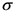.
The surface charge density outside the sphere is,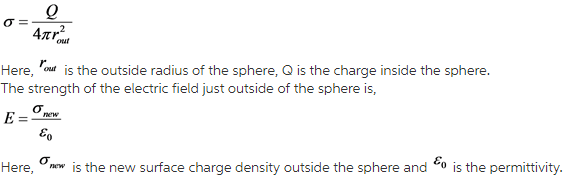Gauss law states that the total electric flux out of a closed surface is equal to the total charge enclosed divided by the permittivity.
State the expression for Gauss law.

(a)
Calculate the surface charge density outside the sphere as follows: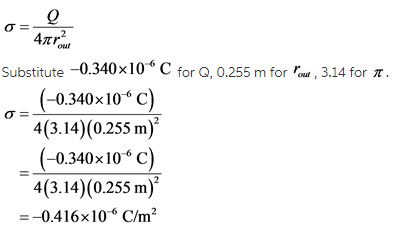Therefore, the surface charge density outside the sphere is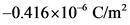.
The new surface charge density outside the sphere as follows: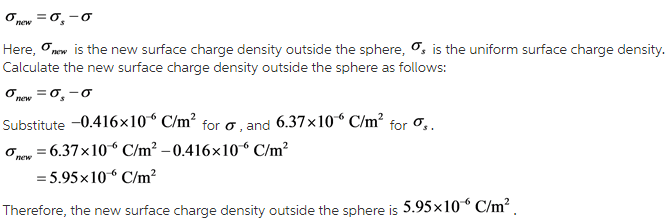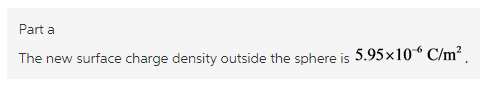Explanation:
Use the formula of surface charge density to calculate the surface charge density outside the sphere. To calculate the new surface charge density outside the sphere takes the difference between uniform surface charge density and surface charge density outside the sphere.

(b)
The strength of the electric field just outside of the sphere is,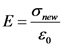Calculate the strength of the electric field just outside of the sphere.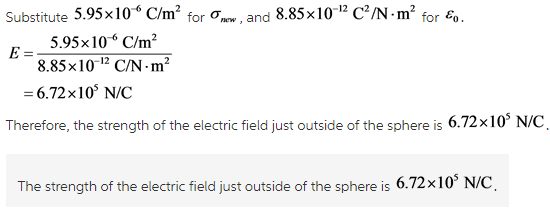Explanation:

( c)
Gauss law states that the total electric flux out of a closed surface is equal to the total charge enclosed divided by the permittivity.
State the expression for Gauss law.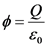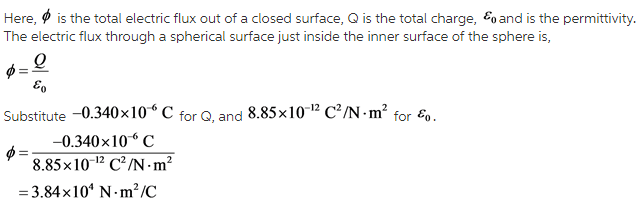Explanation: